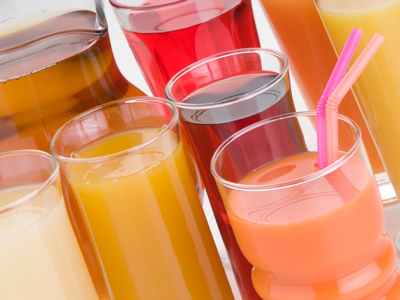Volume can be measured in litres or pints.

# Measures 2 (Easy)

Welcome to the second of our easy maths quizzes on measures. It will help you practice converting metric and imperial units which will set you in good stead for those all-important Eleven Plus exams.

Here’s an approximate list of the metric equivalent of some imperial units:

• 1 yard = 0.91 metres
• 1 mile = 1.61 kilometres
• 1 acre = 0.4 hectares
• 1 pound = 0.45 kilograms
• 1 pint = 0.57 litres

Try to memorise that information if you can – it’ll be a great help in later life. And now, on with the quiz. Good luck!

1.
If 1 mile = 1.61 kilometres, how many miles is 32.2 km?
51 miles
20 miles
48 miles
16 miles
To convert km to miles, divide by 1.61: 32.2 ÷ 1.61 = 20
2.
There are 8 pints in 1 gallon. If 1 pint = 0.57 litres, how many litres are there in 1 gallon?
14.03 litres
4.56 litres
8.57 litres
7.43 litres
To convert pints to litres, multiply by 0.57: 8 x 0.57 = 4.56
3.
If 1 pound = 0.45 kilograms, how many grams are there in 2 pounds?
444 g
900 g
4,444 g
90 g
If 1 pound = 0.45 kg, then 2 pounds = 0.9 kg. We know that there are 1,000 grams in a kg, so 0.9 kg = 900 grams
4.
An estate owner has 480 acres of land. If 1 acre = 0.4 hectares, how many hectares does the estate owner have?
192 hectares
1,200 hectares
220 hectares
240 hectares
To convert acres into hectares, multiply by 0.4: 480 x 0.4 = 192
5.
100 metres is the distance of a sprint race. If 1 yard = 0.91 metres, how many yards are there in 100 metres, to the nearest yard?
91 yards
97 yards
110 yards
112 yards
To convert metres into yards, divide by 0.91: 100 ÷ 0.91 = 109.89, which we round up to 110
6.
There are 14 pounds in 1 stone. If 1 pound = 0.45 kilograms, how many kilograms are there in 1 stone?
6.3 kg
31.1 kg
7 kg
5.8 kg
To convert pounds to kg, multiply by 0.45: 14 x 0.45 = 6.3
7.
A floor measures 5 yards by 6 yards. If 1 yard² = 0.84 m², what is the area of the floor in m²?
47 m²
35.7 m²
15.6 m²
25.2 m²
Area = length x width: 5 x 6 = 30.
Next, convert yards² to m² by multiplying by 0.84: 30 x 0.84 = 25.2.
Be sure you use ² if the number is squared
8.
The distance from London to Edinburgh is 649 km. If 1 mile = 1.61 km, how many miles is it from London to Edinburgh, to the nearest mile?
1,045 miles
642 miles
376 miles
403 miles
To convert km into miles, divide by 1.61: 649 ÷ 1.61 = 403.105, which we round down to 403
9.
A cuboid has a length of 8 cm, a width of 5 cm and a height of 7 cm. What is the volume of the cuboid?
20 cm²
20 cm³
280 cm³
280 cm²
Volume = length × width × height = 8 × 5 × 7 = 280 cm³. Make sure that for area you use square (²) and that for volume you use cube (³)
10.
Pop usually comes in 2 litre bottles. If 1 pint = 0.57 litres, how many pints are there in 2 litres, to 1 decimal place?
1.1 pints
2.4 pints
3.5 pints
4.2 pints
To convert litres into pints, divide by 0.57: 2 ÷ 0.57 = 3.508, which we round down to 3.5
Author:  Frank Evans